Next: Adiabatic Invariants Up: Charged Particle Motion Previous: Invariance of Magnetic Moment

# Poincaré Invariants

An adiabatic invariant is an approximation to a more fundamental type of invariant known as a Poincaré invariant. A Poincaré invariant takes the form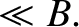(102)

where all points on the closed curve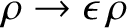in phase-space move according to the equations of motion.

In order to demonstrate thatis a constant of the motion, we introduce a periodic variableparameterizing the points on the curve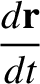. The coordinates of a general point onare thus written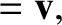and. The rate of change ofis then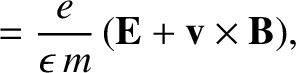(103)

We integrate the first term by parts, and then used Hamilton's equations of motion to simplify the result. We obtain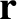(104)

where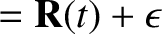is the Hamiltonian for the motion. The integrand is now seen to be the total derivative of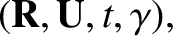along. Since the Hamiltonian is a single-valued function, it follows that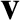(105)

Thus,is indeed a constant of the motion.Next: Adiabatic Invariants Up: Charged Particle Motion Previous: Invariance of Magnetic Moment
Richard Fitzpatrick 2011-03-31